# LessonMaking the Connection

(0 Ratings)

### Quick Look

Grade Level: 11 (9-12)

Time Required: 1 hour

Lesson Dependency: None

Subject Areas: Computer Science, Data Analysis and Probability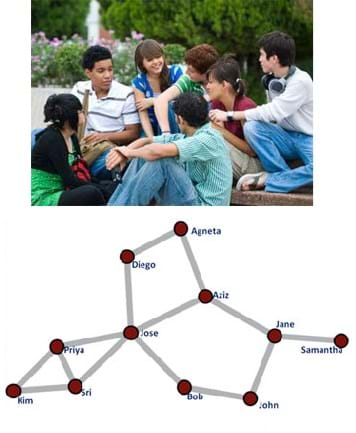A graph shows a simple social network—the social connections of 11 people.copyrightCopyright © State of Connecticut (top), 2011 ADuvvuru, Wikimedia Commons {PD} (bottom) http://www.ct.gov/dcf/cwp/view.asp?a=3622&Q=432386 http://commons.wikimedia.org/wiki/File:Katz_example_net.png

### Summary

Graph theory is a visual way to represent relationships between objects. One of the simplest uses of graph theory is a family tree that shows how different people are related. Another application is social networks like Facebook, where a network of "friends" and their "friends" can be represented using graphs. Students learn and apply concepts and methods of graph theory to analyze data for different relationships such as friendships and physical proximity. They are asked about relationships between people and how those relationships can be illustrated. As part of the lesson, students are challenged to find the social graph of their friends. This prepares students for the associated activity during which they simulate and analyze the spread of disease using graph theory by assuming close proximity to an infected individual causes the disease to spread.

### Engineering Connection

Graph theory is the study of graphs in computer science and mathematics. A graph is a mathematical structure used to model relationships between the objects in a set of objects. In this context, graphs have vertices or "nodes" and a group of edges, which connect pairs of vertices. In computer science, software engineers use graphs to represent communication networks, data organization, computational devices, the flow of computation, and more. On social network websites like Facebook, software engineers exploit graph theory to market products. They analyze your "friends" and if a particular ad is relevant to you (you clicked on it), the ad shows up on the pages of your friends who have similar interests. By using content on your page and your friends' pages, content advertising is targeted to users who are most likely to buy particular products.

### Learning Objectives

After this lesson, students should be able to:

• Explain the basic concepts of graph theory.
• Apply graph theory to the analysis of a network.

### Educational Standards Each TeachEngineering lesson or activity is correlated to one or more K-12 science, technology, engineering or math (STEM) educational standards. All 100,000+ K-12 STEM standards covered in TeachEngineering are collected, maintained and packaged by the Achievement Standards Network (ASN), a project of D2L (www.achievementstandards.org). In the ASN, standards are hierarchically structured: first by source; e.g., by state; within source by type; e.g., science or mathematics; within type by subtype, then by grade, etc.

###### Common Core State Standards - Math
• (+) Use matrices to represent and manipulate data, e.g., to represent payoffs or incidence relationships in a network. (Grades 9 - 12) More Details

Do you agree with this alignment?

• Graph functions expressed symbolically and show key features of the graph, by hand in simple cases and using technology for more complicated cases. (Grades 9 - 12) More Details

Do you agree with this alignment?

###### International Technology and Engineering Educators Association - Technology
• Use various approaches to communicate processes and procedures for using, maintaining, and assessing technological products and systems. (Grades 9 - 12) More Details

Do you agree with this alignment?

###### National Council of Teachers of Mathematics - Math
• create and use representations to organize, record, and communicate mathematical ideas (Grades Pre-K - 12) More Details

Do you agree with this alignment?

• use representations to model and interpret physical, social, and mathematical phenomena (Grades Pre-K - 12) More Details

Do you agree with this alignment?

###### National Science Education Standards - Science
• Use technology and mathematics to improve investigations and communications. A variety of technologies, such as hand tools, measuring instruments, and calculators, should be an integral component of scientific investigations. The use of computers for the collection, analysis, and display of data is also a part of this standard. Mathematics plays an essential role in all aspects of an inquiry. For example, measurement is used for posing questions, formulas are used for developing explanations, and charts and graphs are used for communicating results. (Grades 9 - 12) More Details

Do you agree with this alignment?

• Scientists rely on technology to enhance the gathering and manipulation of data. New techniques and tools provide new evidence to guide inquiry and new methods to gather data, thereby contributing to the advance of science. The accuracy and precision of the data, and therefore the quality of the exploration, depends on the technology used. (Grades 9 - 12) More Details

Do you agree with this alignment?

• Scientists conduct investigations for a wide variety of reasons. For example, they may wish to discover new aspects of the natural world, explain recently observed phenomena, or test the conclusions of prior investigations or the predictions of current theories. (Grades 9 - 12) More Details

Do you agree with this alignment?

• Mathematics is essential in scientific inquiry. Mathematical tools and models guide and improve the posing of questions, gathering data, constructing explanations and communicating results. (Grades 9 - 12) More Details

Do you agree with this alignment?

###### Nebraska - Science
• Use tools and technology to make detailed qualitative and quantitative observations (Grades 9 - 12) More Details

Do you agree with this alignment?

• Analyze and interpret data, synthesize ideas, formulate and evaluate models, and clarify concepts and explanations (Grades 9 - 12) More Details

Do you agree with this alignment?

Suggest an alignment not listed above

### Subscribe

Get the inside scoop on all things TeachEngineering such as new site features, curriculum updates, video releases, and more by signing up for our newsletter!
PS: We do not share personal information or emails with anyone.

### More Curriculum Like This

High School LessonGraph Theory in Drama

Students use graph theory to create social graphs for their own social networks and apply what learn to create a graph representing the social dynamics found in a dramatic text.

High School LessonSets-Nodes-Edges: Representing Complex Networks in Graph Theory

Students learn about complex networks and how to represent them using graphs. They also learn that graph theory is a useful mathematical tool for studying complex networks in diverse applications of science and engineering, such as neural networks in the brain, biochemical reaction networks in cells...

High School ActivityGraphing the Spread of Disease

Students simulate disease transmission by collecting data based on their proximity to other students. Students learn real-world engineering applications of graph theory and see how numerous instances of real-world relationships can be more thoroughly understood by applying graph theory.

High School ActivityGraphing Your Social Network

Students analyze their social networks using graph theory. They gather data on their own social relationships, either from Facebook interactions or the interactions they have throughout the course of a day, recording it in Microsoft Excel and using Cytoscape (a free, downloadable application) to gen...

### Pre-Req Knowledge

The teacher should have an understanding of graph theory and its basic concepts. Students learn about the concepts of graph theory during the lesson.

### Introduction/Motivation

In this lesson, you are going to explore the concepts of graph theory as it applies to relationships in social networks. What is a social network? For instance: you, your siblings, your parents, grandparents and the rest of your family are a social network that could be represented in a graph called a family tree. Software and network engineers use graph theory to represent the relationships present in communication networks and how different parts of computer programs interact with each other. The designers of Facebook (who are software engineers) use graph theory to map your social connections and then use that information to target the ads that you see on your page.

To begin the lesson, consider the relationships that connect people in social graphs. To prepare you for the study of basic graph theory, you will be asked about the relationships between people and how those relationships can be illustrated. We will also talk about the importance and value of mapping relationships, which is how Facebook uses the social graph of members to focus marketing for their advertisers. (Use the questions below to help guide a class discussion.)

• What are some examples of relationships that connect people? (Answer: Friends, family, teams and clubs.)
• How can relationships between people be organized or illustrated for ease in understanding? (Answers: List, outline, vertex and edge graph.)
• What is a graph? (Expected answers: X-Y graph, bar graph, histogram, box and whisker plot, pie chart, etc.)
• What is the Facebook social graph? (Answer: The connections that exist between people through friendships.)
• How could advertisers use the Facebook social graph in their marketing of products? (Answer: Advertisers find products your friends like and market those same items to you.)

Next, you will create and use social graphs to help visualize the relationships between people. You will complete these tasks: 1) create a social graph of your friends, 2) use the internet to research basic graph theory concepts, and 3) get an understanding of six degrees of separation.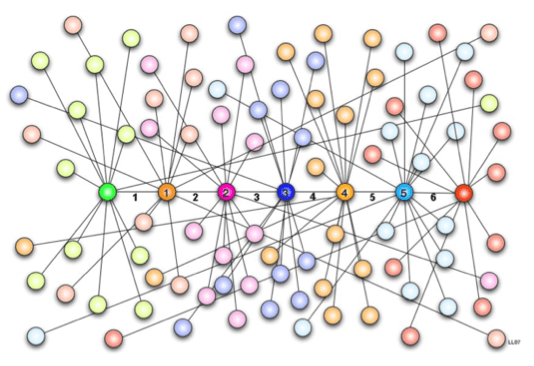This graph shows the concept of six degrees of separation, the idea that everyone is no more than six "steps" away from each person on Earth.copyrightCopyright © 2007 Laurens van Lieshout, Wikimedia Commons {PD} http://commons.wikimedia.org/wiki/File:Six_degrees_of_separation_01.png

To begin your exploration of social graphs and graph theory, you need to find the social graph of your friends. To do this, you will make a list of five of your friends, and then ask each of those friends to make a list of five of their friends. Using this data along with some research, you will construct a social graph of the results.

(Give students one minute to write a list of five friends in the class. Then give them 10 minutes total to find those friends in class and copy each of their friend lists. Later, they will make social graphs with these social network lists. Alternatively, assign students to do this data gathering outside of class time, not necessarily using friends in the class.)

Next, you will research the basic concepts of graph theory and the ideas surrounding six degrees of separation. As you research, be sure to learn what a social graph looks like so that you can draw one later using the friend information you collected. Here are some suggested vocabulary terms to help with your research (write the terms on the classroom board or make sure students write them down for future reference): six degrees of separation, graph theory, node, vertex, child vertex, sibling vertex, edge (edge graph), depth first search (DFS), and breadth first search (BFS). Make sure you understand these terms.

(Note to the teacher: This research assignment is intentionally left open ended. Direct students to explore internet resources to learn about graph theory and the vocabulary words, which are also defined in the Vocabulary/Definitions section.)

Next, construct a social graph for your friends network. Either do this by hand or use a tool like yEd or Dia (see the Additional Multimedia Support section). Once created, describe your graphs using the new vocabulary terms, such as vertex (or node) and edges, and apply a degree of separation analysis to the graph. That is, how far away are you from each other via the connections on your graph? Also, create a vertex list, adjacency matrix and adjacency list for your graph.

After you have completed this graph theory research and practice project, we'll conduct the associated activity Graphing the Spread of Disease. During this activity, you will collect data that models disease transmission and test your new skills and graph theory knowledge to make a graph of the simulation, which you will analyze using depth first search and breadth search first algorithms.

### Lesson Background and Concepts for TeachersFigure 1. An example regular graph from graph theory. This graph has four vertices and connecting edges, and each vertex has two edges leading from it.copyrightCopyright © 2013 Steve Hamersky, University of Nebraska-Omaha

Graph Theory

Graph theory is the mathematical study of graphs. A graph consists of a set or collection of vertices or nodes (in students' social graphs, these are the people) and a set or collection of edges (in students' social graphs, these are the friendships) that connect the vertices. As an illustration, a graph defined by vertex set V = {1, 2, 3, 4} and edge set E = {(1, 3), (1, 4), (2, 3), (2, 4)} would have the diagram shown in Figure 1. Vertices that are connected by an edge are said to be adjacent. The degree of a vertex is the number of edges connecting to the vertex as an end point. In Figure 1, all vertices have a degree of two. In graph theory, two of the different types of graphs are the complete graph and the regular graph. A complete graph is a graph where every vertex is adjacent to every other vertex. A regular graph has the same degree on all vertices (such as the Figure 1 graph, in which each node/vertex has two edges leading to it.)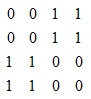Figure 2. The adjacency matrix for the Figure 1 graph.

An adjacency matrix for a graph with n vertices is an n x n matrix, where entry (i, j) is 1 if there is an edge in the graph between vertex i and j; otherwise the entry is 0. Every vertex must be numbered so that an adjacency matrix can be created. Figure 2 shows the adjacency matrix for the Figure 1 graph.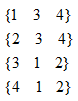Figure 3. The adjacency list for the Figure 1 graph.

An adjacency list for a graph has a row for each vertex and contains the vertex label followed by the vertex labels of adjacent vertices. Every vertex must be numbered so that an adjacency list can be created. The first number in the row is the vertex and the other numbers are other vertices connected to the first number via an edge. Figure 3 shows the adjacency list for the Figure 1 graph.

A path in a graph from vertex v to u is a sequence of edges where the first edge starts at v, each successive edge connects to the previous edge, and the final edge terminates at u. A path from 1 to 2 in the Figure 1 graph would be: (1, 3), (3, 2). A vertex v is reachable from vertex u, if there is a path from v to u. A graph is connected if every vertex is reachable from every other vertex; otherwise the graph is considered disconnected.

A graph traversal is the process of visiting every vertex of a graph. A number of algorithms or methods of graph traversal exist, with the most common being depth first search (DFS) and breadth first search (BFS). Considering a given node as the parent and connected nodes as children, DFS visits the child vertices before visiting siblings, whereas BFS visits the sibling vertices before the child vertices. For example see Figure 4.

DFS and BFS have their own special applications. For example, DFS is useful in creating or solving mazes (see Figure 5), while BFS is more useful in finding winning game strategies (see Figure 6). Refer to the DFS and BFS Algorithms Instructions for how to apply DFS and BFS algorithms.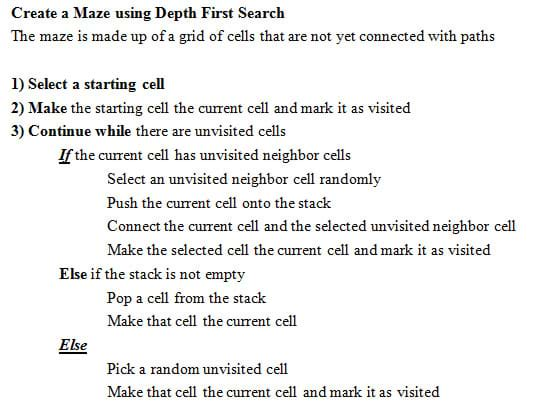Figure 5. An example of how to create a maze using a DFS strategy.copyrightCopyright © 2013 Steve Hamersky, University of Nebraska-Omaha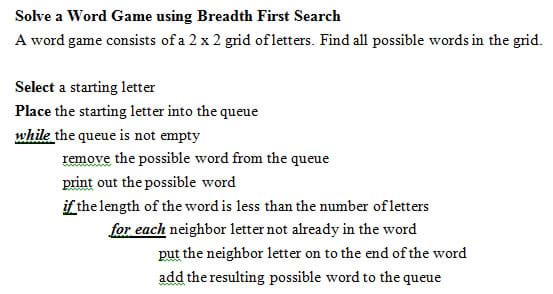Figure 6. An example of how to solve a word game using a BFS strategy.copyrightCopyright © 2013 Steve Hamersky, University of Nebraska-Omaha

For this grid of letters:

Using the breadth first search algorithm and starting with letter A produces the following:

Teacher and Student Resources

See the Additional Multimedia Support section for a list of recommended resources.

### Associated Activities

• Graphing the Spread of Disease - Students simulate disease transmission by collecting data based on their proximity to other students. They do this by having Bluetooth devices "discover" each other (or by hand, using no technology) with the assumption that close proximity to an infected person causes the disease to spread. Then students apply graph theory to analyze the data.

### Vocabulary/Definitions

adjacency list: An adjacency list for a graph has a row for each vertex and contains the vertex label followed by the vertex labels of adjacent vertices.

adjacency matrix: An adjacency matrix for a graph with n vertices is an n x n matrix where each entry (i, j) is a 1 if there is an edge in the graph between vertex i and j; otherwise the entry is 0.

breadth first search (BFS): An algorithm or method of graph traversal that considers a given node as the parent and connected nodes as children; BFS visits the sibling vertices before the child vertices.

child vertex: Nodes in a graph that are directly connected to the current node. If the first node (parent node) is selected, then one of the nodes connected to it is the child node. That child node then becomes a parent node, and a node connected to it becomes a child node.

depth first search (DFS): An algorithm or method of graph traversal that considers a given node as the parent and connected nodes as children; DFS visits the child vertices before visiting sibling vertices.

edge (edge graph): A connection between nodes.

graph theory: The study of mathematical structures (graphs) so as to model relationships between objects from a collection.

sibling vertex: Nodes in a graph that are on the same level as the child node, connected to the same parent node with a single edge. Sibling nodes may or may not be connected to each other.

six degrees of separation: The theory that everyone is six or fewer steps away from knowing any other person in the world. This theory implies that a chain of "friend to friend" can be made to connect any two people in the world in a maximum of six steps.

vertex list: A listing of all the vertices present in a graph.

vertex or node: The fundamental unit of which graphs are formed. Plural: vertices.

### Assessment

Formative Assessment: As students are engaged in the lesson research assignments, observe them and ask questions to evaluate their comprehension of the following expectations:

• Are students applying the basic vocabulary of graph theory, such as vertex and edge? (Through questioning and interactions, reinforce their correct use of the vocabulary terms.)
• Can students translate between the adjacency matrix or list and the graph?
• Do students understand how graphs can be used to represent relationships present in a collection of data?

Summative Assessment: At lesson end, administer the Making the Connection Lesson Assessment, which is composed of four writing and performance assessment questions. Review students' answers to gauge the effectiveness of their research and their resulting comprehension of the topic.

### Additional Multimedia Support

Recommended teacher and student resources:

• Graph Theory Lessons: These Java Web Start programs illustrate graph theory concepts. http://www.mathcove.net/petersen/lessons/
• Cytoscape: An open source platform for data visualization. http://www.cytoscape.org/
• yEd: A powerful desktop application that can be used to quickly and effectively generate high-quality diagrams. You can run the application from the computer or web-based. http://www.yworks.com
• Dia: A free diagram creation program available in most OS platforms. http://dia-installer.de/

© 2013 by Regents of the University of Colorado; original © 2013 Board of Regents, University of Nebraska

### Contributors

Steve Hamersky, Brian Sandall

### Supporting Program

IMPART RET Program, College of Information Science & Technology, University of Nebraska-Omaha

### Acknowledgements

The contents of this digital library curriculum were developed as a part of the RET in Engineering and Computer Science Site on Infusing Mobile Platform Applied Research into Teaching (IMPART) Program at the University of Nebraska-Omaha under National Science Foundation RET grant number CNS 1201136. However, these contents do not necessarily represent the policies of the NSF and you should not assume endorsement by the federal government.